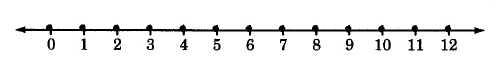How can I solve for y=6+2?

Are you trying to find the value for y?

All you need to do is just add those two numbers together. 6 + 2 = 8.

This number line should help:Our first number is 6, so start from there. Now we have to add 2, meaning we go 2 places to the right. After one place, we get 7, and after two, we get 8.

Therefore, y = 8.

thanked the writer.